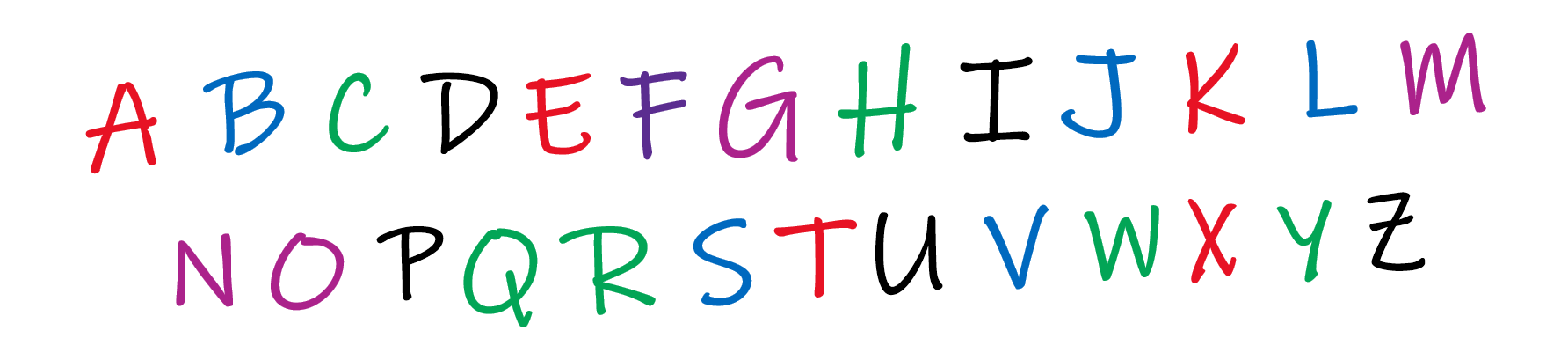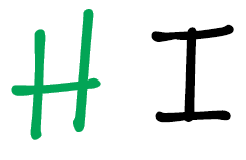Home Theory What is the Big O notation? [Simplified]

# What is the Big O notation? [Simplified]

0 comment

As soon as you hear the word ‘Big O’, does your mind go “Oh my god! Oh no! This again?” The next thing following it would be time complexity and what not. Suddenly, your brain starts getting this confusing signals and you lose track of what is happening.

If you have felt this at some point of time while learning programming, then I want to tell you that you are not alone. In fact, you are proceeding in the right direction. But all these notations can start to get overwhelming in the beginning. With this article I hope to end all your worries and this fear will become a thing of the past.

#### What is Big O?

The Big O notation is used to represent the time complexity of an algorithm. That means, it determines the rate of growth. It is an important metric as it helps to estimate how long our algorithm will execute.

It simply means what will happen in a case when everything goes wrong. Think like this, you are the manager of a football team and you want to calculate the winning odds. Sure, you can include your best players in those calculations, but to calculate the Big O complexity, you need to assume that all your best players are injured, and you have to play with the remaining lot.

Similarly, in computer programming, if you are writing a code to sort some numbers, the worst possible case could be when the numbers you obtain are sorted in the reverse order.

So, let us define some of the commonly used terms in Big O notation, and in each of the case, we will look at the worst possible case.

We can understand the Big O notation as a kid. Do you remember how we play with letters when we are trying to learn the English alphabet.

Let’s say that you have all these alphabets lying around over here.

##### What is O(1) ?

Suppose that I ask you, just go and pick any character you like. This is completely your choice which character you pick, and you can do this no matter how many characters are lying on the floor. Right now, there are 26 characters, but no matter how many objects you have, you can still pick up any random character. This is what an O(1) time complexity looks like.

An algorithm that follows O(1) time complexity is independent of the input size, and will perform with the same efficiency in every scenario.

Example: You want to add an element to a Queue. No matter how long the queue is, adding an element would take O(1) time.

##### What is O(n) ?

Go back to the same pile of characters lying on the floor. I now ask you to get me a specific letter ‘K’. You go about looking for the letter ‘K’ in the mess on the floor. Remember that we are considering the worst case scenario. You start to look at each of the alphabet. In the worst case, you will find ‘K’ at the very end. That means you had to look through a total of 26 characters.

Let us say now, I add digits also to this pile. You now have to look through (26 + 10) = 36 elements.

This is what the O(n) time complexity looks like. n refers to the input size here. So, an algorithm which follows the time complexity of O(n) would do at most n primitive operations before giving you the result.

Example: Checking if a string is a palindrome. You need to go over each character to verify if it is true. If the string is 10 characters, you need 10 comparisons. If the string is 10,000 characters, you would need at most 10000 comparisons. Means we are growing at the rate of O(n).

##### What is O(n^2) ?

For one more time, let us go back to the pile of characters on the floor. I now ask you to sort the entire list of characters. Remember that you are taking in account the worst case.

• You look through all the 26 characters, to find the letter ‘A’
• Then you look through all the remaining 25 characters, to find the letter ‘B’
• Since, we are considering the worst case, you again look through all the 24 characters, to find the letter ‘C’

Hence, the total number of look-ups you are doing is
26 + 25 + 24 +23 + ..... 1

Or if you consider 26 to be the input size, or n, we can also write this as:

n + (n-1) + (n-2) + (n -3) + .....

If we try to solve this:

n + (n-1) + (n -2) + (n -3) + ..... + 1
\newline
= n + n +n ....  (n-times) - (1 + 2 + 3 + ..... n)
\newline
= n * n - (\sum n)
\newline
= n^2 - n

This means that you are doing a look up equivalent to n^2 times. Hence, this kind of a time complexity is called O(n^2).

##### What is O(n \log n)?

If you made it this far, this part is gonna be easy as well. Now you have a sorted list of alphabets, which means that you can know which character will come after which one.

I think of a character, and I ask you to guess it. Instead of making random guesses, you can take a smart approach.

YOU:
I have these characters.So, is the character you guessed comes after M(mid) or before it?

ME:
It comes before M.

YOU:
This means I can discard all the characters after M.Now, tell me does your character come after or before G (remaining mid)?

ME:
It comes after G.

YOU:
Interesting, I can again discard all the characters before G. I am remaining with.Does it come after J or before J(mid again).

ME:
Before J.

YOU:
Cool, I am only left withIs your letter H?

ME:
Yes.

So you see, how we were able to exactly half our search space after every iteration? Let us look at it mathematically. Assuming that there are n characters and you find the solution after a total of k half operations.

n * (\frac{1}{2}) ^ k = 1
\newline or \newline
n * \frac{1}{2^k} = 1
\newline or \newline
n = 2 ^ k
\newline or \newline
\log_2 n = k

This gives you a time complexity of O(\log n).

#### Final Thoughts:

I hope these examples gave you a good explanation of how the Big O time complexity actually works. We just count the number of primitive operations we would be doing in the worst case. If this thought is clear in your mind, then it shouldn’t be a problem to think about O(n!) and other time complexities.

0 comment

#### You may also like

This site uses Akismet to reduce spam. Learn how your comment data is processed.

This website uses cookies to improve your experience. We'll assume you're ok with this, but you can opt-out if you wish. Accept Read More